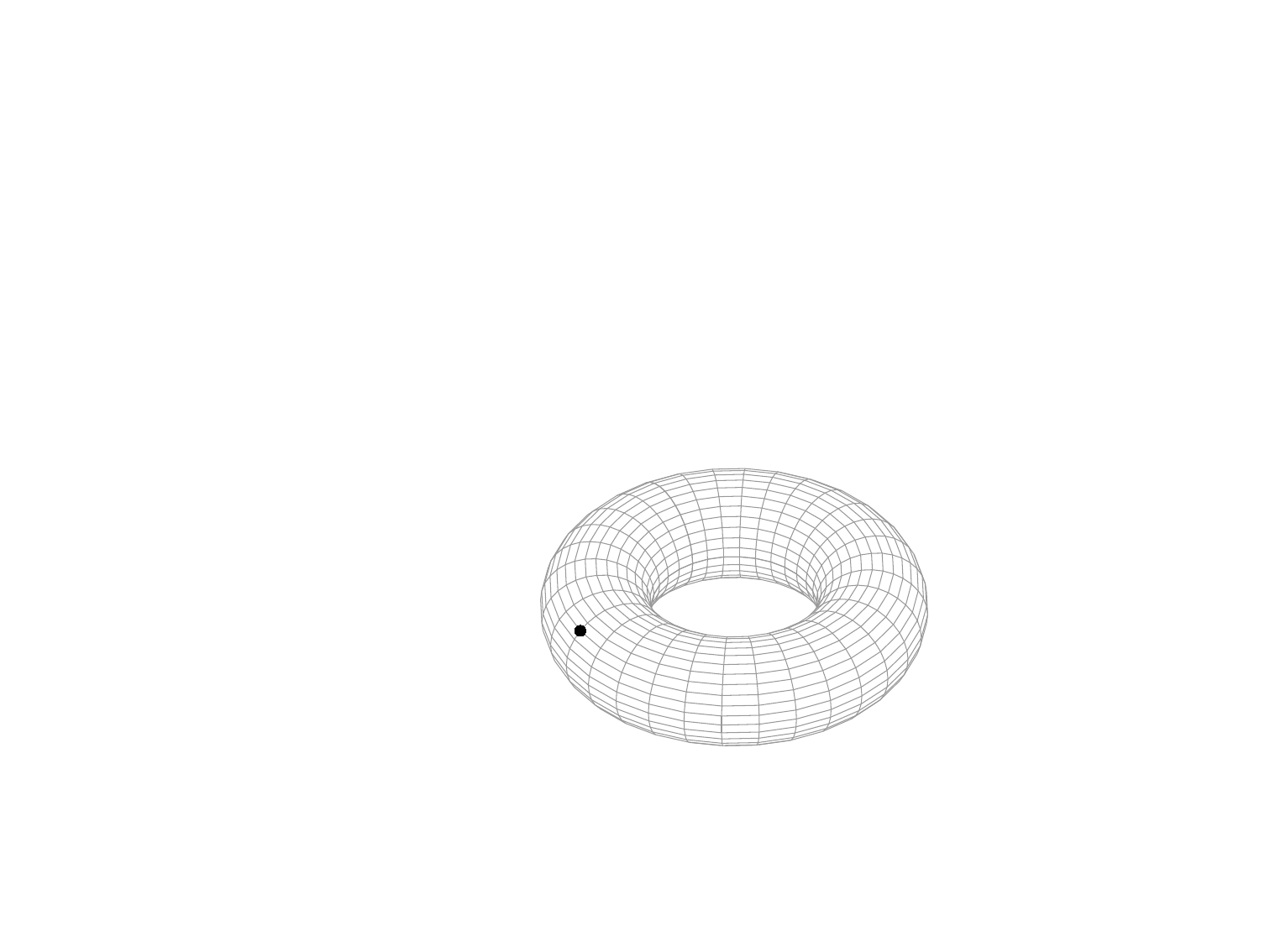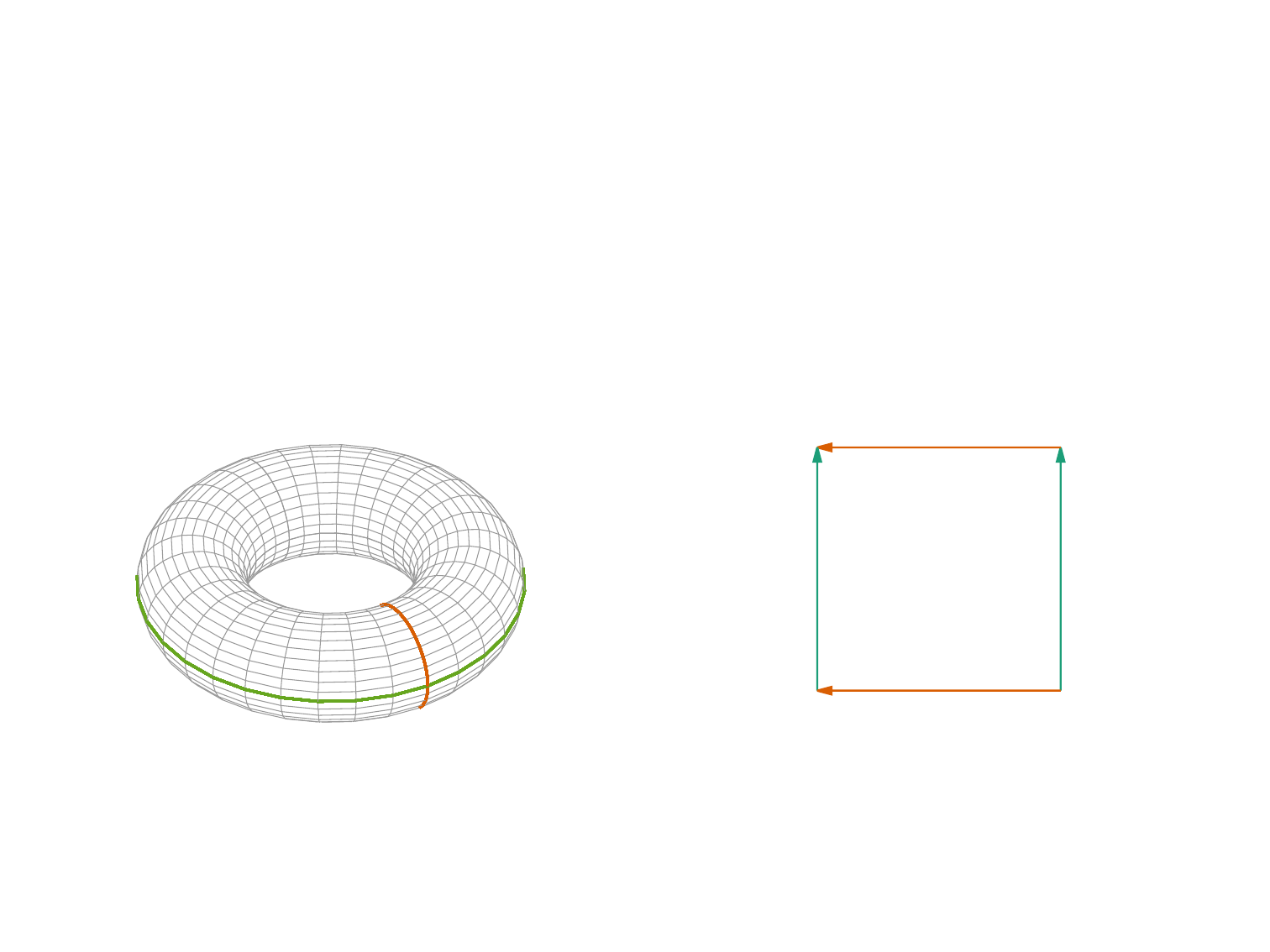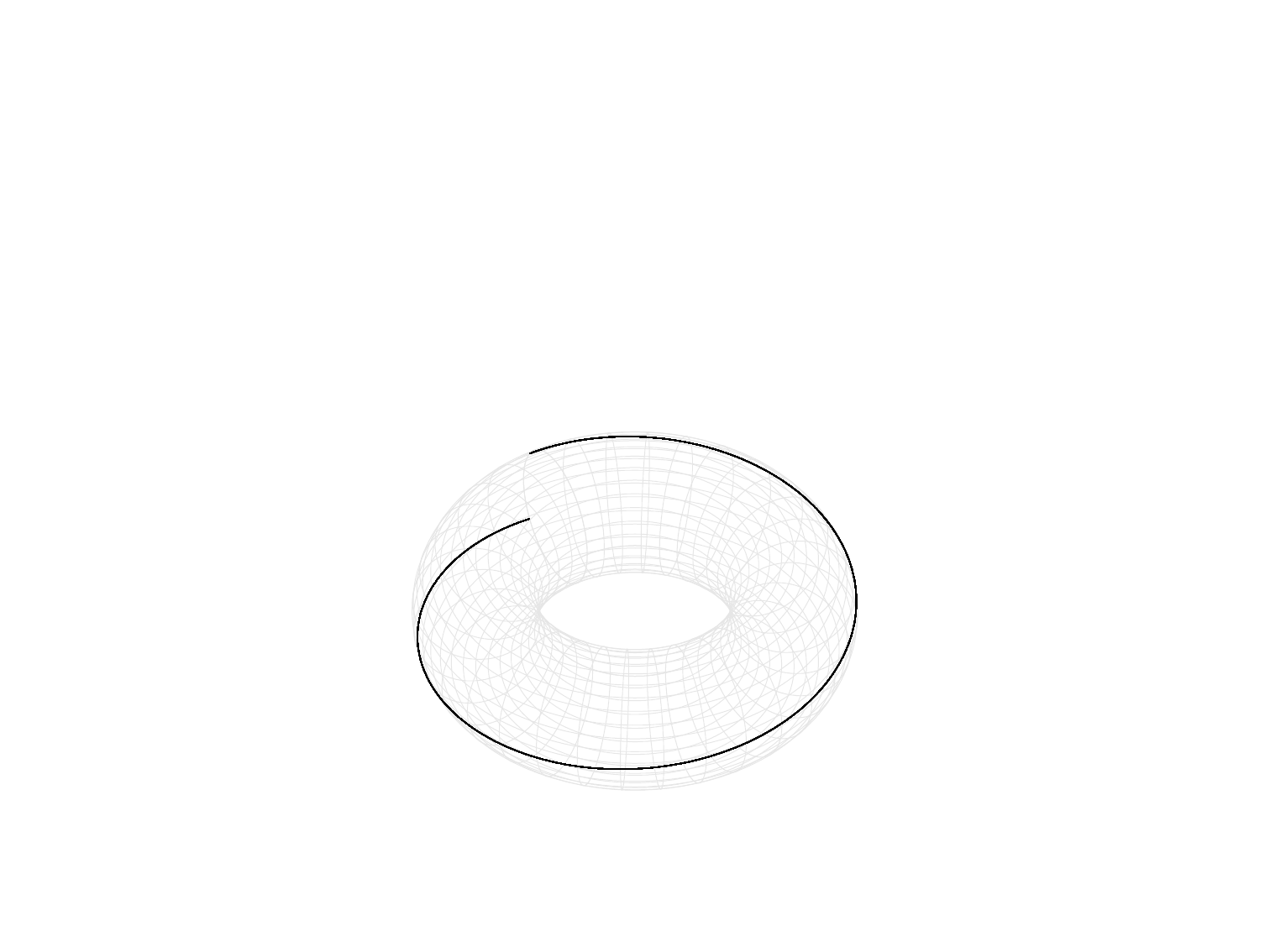Ergodic dynamical systems
Juan M. Bello-Rivas
jmb@ices.utexas.eduOutline
1 Recap
2 ErgodicityOutline
1 Recap
2 ErgodicityMolecular Dynamics is (mostly) about computing integrals like
Z
X
f(x) π(x) dx,
where X R
n
.(a) If n is small (i.e., n < 7), then quadrature formulas suﬃce.
(b) Otherwise, we need other techniques: Monte Carlo and
variants.In essence, Monte Carlo is about noticing that
Z
X
f(x) π(x) dx = E[f]
and then using the Central Limit Theorem:
E[f] =
1
N
N
X
n=1
f(x
i
) + Error(N )
where the x
i
are random samples of the probability µ with p.d.f.
π(x) and
Error(N) Normal(0,
1
N
Var(f)
| {z }
variance
) as N .The problem then shifts to obtaining random samples of µ. One
possible avenue is to use the Metropolis algorithm:
Markov chain T
p.d.f. π of µ
)
sample paths from a Markov chain A,
where A is s.t. lim
n→∞
A
n
(x, ·) = π(·).Outline
1 Recap
2 ErgodicityIntroduction
Ergodicity in a nutshell: Let t [0, ) 7→ x(t) X , then
lim
T →∞
1
T
Z
T
0
f(x(t)) dt =
Z
X
f(x) π(x) dx.
This yields another approach to sampling.
How is it even possible? The integrand in the LHS is 1-dimensional
whereas the one in the RHS is n-dimensional!Introduction
Space-ﬁlling curveDynamical systems
Let X be a set that we will refer to as the state space.
Example
Let X be the two-dimensional torus. A point in X corresponds to
a pair of angles, (x
1
, x
2
), determining a point on the torus.
(x
1
, x
2
)Dynamical systems
Let µ be a probability measure deﬁned on X . This means that to
each C X we can assign a value µ(C) between 0 and 1
Example
x
2
x
1Dynamical systems
Example (cont.)
x
2
x
1
C
Let C be a (measurable) subset of the torus. We deﬁne
µ(C) =
x
C
dx
1
dx
2
4π
2
.Dynamical systems
Example (Flow on the torus)
Let x = (x
1
, x
2
) [0, 2π) × [0, 2π) and ω = (ω
1
, ω
2
) R
2
.
Consider the trajectories ϕ
t
(x) = x + t ω of a free particle started
at x with constant velocity ω.Dynamical systems
In general, we may consider a family of mappings ϕ
t
: X X
parameterized by t 0 so that, for all x X , we have
ϕ
0
(x) = x
and
ϕ
t+s
(x) = ϕ
s
(ϕ
t
(x)).Dynamical systems
Deﬁnition
A dynamical system is a tuple (X , µ, ϕ).
Example
X = [0, 2π) × [0, 2π), µ(C) =
x
C
dx
1
dx
2
4π
2
, ϕ
t
(x) = x + .Dynamical systems
Example (Microcanonical ensemble)
Let X = R
2N
, H : X R, and
µ(C) =
R
C
δ(H(x) E)dx
R
X
δ(H(x
0
) E)dx
0
,
where x = (q
0
, p
0
) X and ϕ
t
(x) is the solution of the system of
ODEs
dq
dt
(t) =
p
H(q(t), p(t)),
dp
dt
(t) = −∇
q
H(q(t), p(t)),
q(0) = q
0
,
p(0) = p
0
.Dynamical systems
Deﬁnition
Let f : X R. The time average of f (starting at x) is
¯
f(x) = lim
T →∞
1
T
Z
T
0
f(ϕ
t
(x)) dt.
Example
For ϕ
t
(x
1
, x
2
) = (x
1
+
1
, x
2
+
2
), we have
¯
f(x
1
, x
2
) = lim
T →∞
1
T
Z
T
0
f(x
1
+
1
, x
2
+
2
) dt.Dynamical systems
Deﬁnition
Denote by π the probability mass (or density) function of the
probability measure µ. The space average of the function f is
hfi =
Z
X
f(x) π(x)dx.
Example
Let X = [0, 2π) × [0, 2π), then for f = f(x, y),
hfi =
2π
Z
0
2π
Z
0
f(x, y)
dx dy
4π
2
.Dynamical systems
Deﬁnition
We say that (t, x) 7→ ϕ
t
(x)
preserves the measure µ for
the set C X if
Z
ϕ
t
(C)
π(x) dx =
Z
C
π(x) dx
for all C X and t 0.
x
2
x
1
C
ϕ
t
(C)Dynamical systems
Deﬁnition
A set C X is said to be invariant if,
ϕ
t
(x) C for all x C and all t 0.
In general, we write ϕ
t
(C) = C.Ergodic theorem
Theorem (Birkhoﬀ-Khinchin (1931))
Consider the dynamical system (X , µ, ϕ) such that ϕ preserves the
measure µ. Let C X be an invariant set and let f : C R be
any integrable function. Then, the limit
¯
f(x) = lim
T →∞
1
T
Z
T
0
f(ϕ
t
(x)) dt
exists almost everywhere in C. Moreover,
¯
f(x) is independent of
the choice of the initial point x (so we write
¯
f from now on).